Next: Elastic and Inelastic Scattering Up: Scattering Theory Previous: Low-Energy Scattering

# Resonant Scattering

There is a significant exception to the energy independence of the scattering cross-section at low incident energies described in the previous section. Suppose that the quantity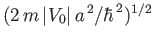is slightly less than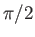. As the incident energy increases,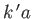, which is given by Equation (10.129), can reach the value. In this case,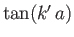becomes infinite, so we can no longer assume that the right-hand side of Equation (10.124) is small. In fact, at the value of the incident energy at which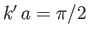, it follows from Equation (10.124) that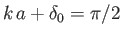, or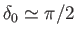(because we are assuming that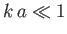). This implies that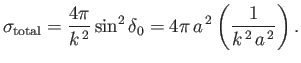(10.130)

Note that the total scattering cross-section now depends on the energy. Furthermore, the magnitude of the cross-section is much larger than that given in Equation (10.128) for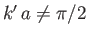(because, whereas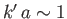).

The origin of the rather strange behavior described in the previous paragraph is easily explained. The condition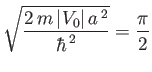(10.131)

is equivalent to the condition that a spherical well of depth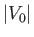possesses a bound state at zero energy. Thus, for a potential well that satisfies the preceding equation, the energy of the scattering system is essentially the same as the energy of the bound state. In this situation, an incident particle would like to form a bound state in the potential well. However, the bound state is not stable, because the system has a small positive energy. Nevertheless, this sort of resonant scattering is best understood as the capture of an incident particle to form a metastable bound state, followed by the decay of the bound state and release of the particle. The cross-section for resonant scattering is generally far higher than that for non-resonant scattering.

We have seen that there is a resonant effect when the phase-shift of the S-wave takes the value. There is nothing special about thepartial wave, so it is reasonable to assume that there is a similar resonance when the phase-shift of theth partial wave is. Suppose that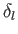attains the valueat the incident energy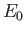, so that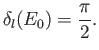(10.132)

Let us expand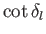in the vicinity of the resonant energy: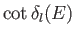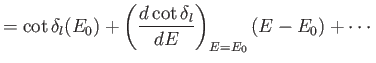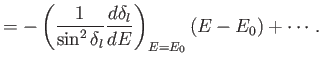(10.133)

Defining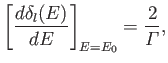(10.134)

we obtain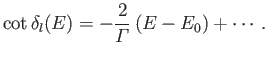(10.135)

Recall, from Equation (10.93), that the contribution of theth partial wave to the total scattering cross-section is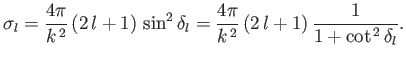(10.136)

Thus,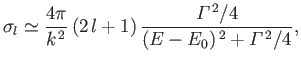(10.137)

which is known as the Breit-Wigner formula . The variation of the partial cross-section,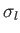, with the incident energy has the form of a classical resonance curve. The quantity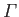is the width of the resonance (in energy). We can interpret the Breit-Wigner formula as describing the absorption of an incident particle to form a metastable state, of energy, and lifetime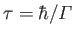.Next: Elastic and Inelastic Scattering Up: Scattering Theory Previous: Low-Energy Scattering
Richard Fitzpatrick 2016-01-22